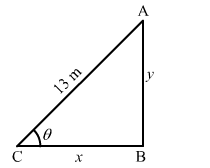# A ladder 13 m long leans against a wall.

Question:

A ladder 13 m long leans against a wall. The foot of the ladder is pulled along the ground away from the wall, at the rate of 1.5 m/sec. How fast is the angle θ between the ladder and the ground is changing when the foot of the ladder is 12 m away from the wall.

Solution:

Let the bottom of the ladder be at a distance of x m from the wall and its top be at a height of y m from the ground.Then,

$\tan \theta=\frac{y}{x}$ and

$x^{2}+y^{2}=(13)^{2}$

$\Rightarrow x^{2}\left(1+\tan ^{2} \theta\right)=169$

$\Rightarrow \sec ^{2} \theta=\frac{169}{x^{2}}$

$\Rightarrow 2 \sec ^{2} \theta \tan \theta \frac{d \theta}{d t}=169\left(\frac{-2}{x^{3}}\right) \frac{d x}{d t}$

$\Rightarrow \frac{d \theta}{d t}=\frac{-338 \times 1.5}{(12)^{3} 2 \sec ^{2} \theta \tan \theta}$             ....(1)

When $x=12, y=\sqrt{169-144}=5 \mathrm{~m}$

So,

$\sec \theta=\frac{13}{12}$ and $\tan \theta=\frac{12}{5}$

From eq. (1), we get

$\frac{d \theta}{d t}=\frac{-338 \times 1.5}{(12)^{3} \times 2 \times\left(\frac{13}{12}\right)^{2} \times \frac{5}{12}}=\frac{-338 \times 1.5}{10 \times 169}=-0.3 \mathrm{rad} / \mathrm{sec}$